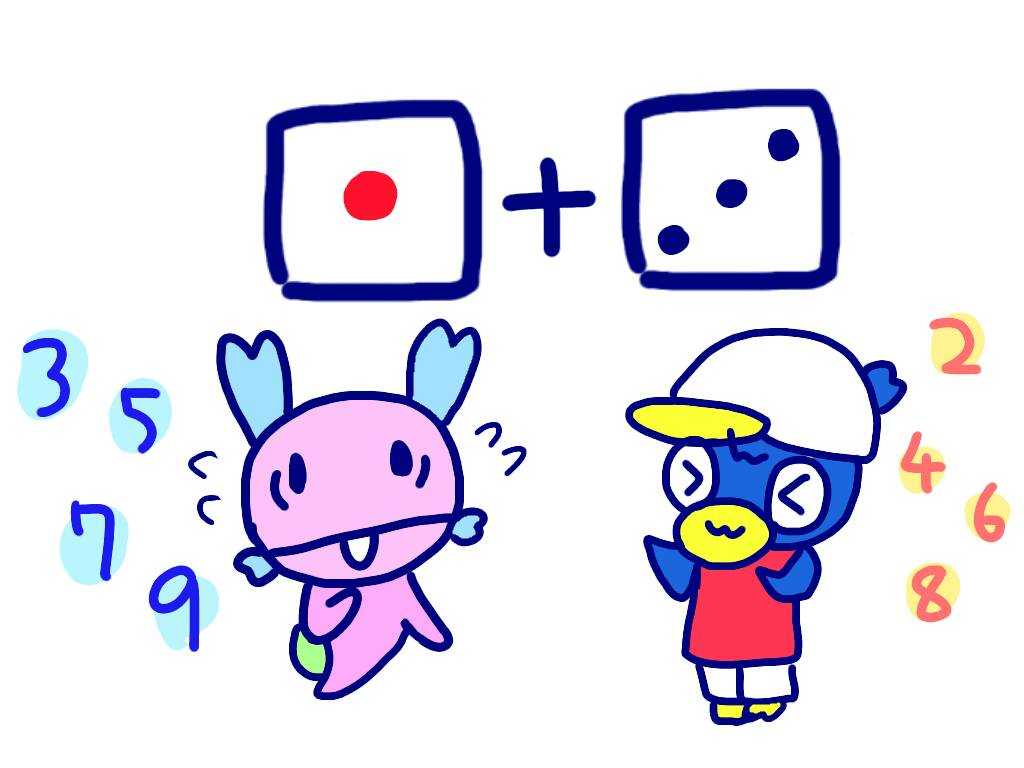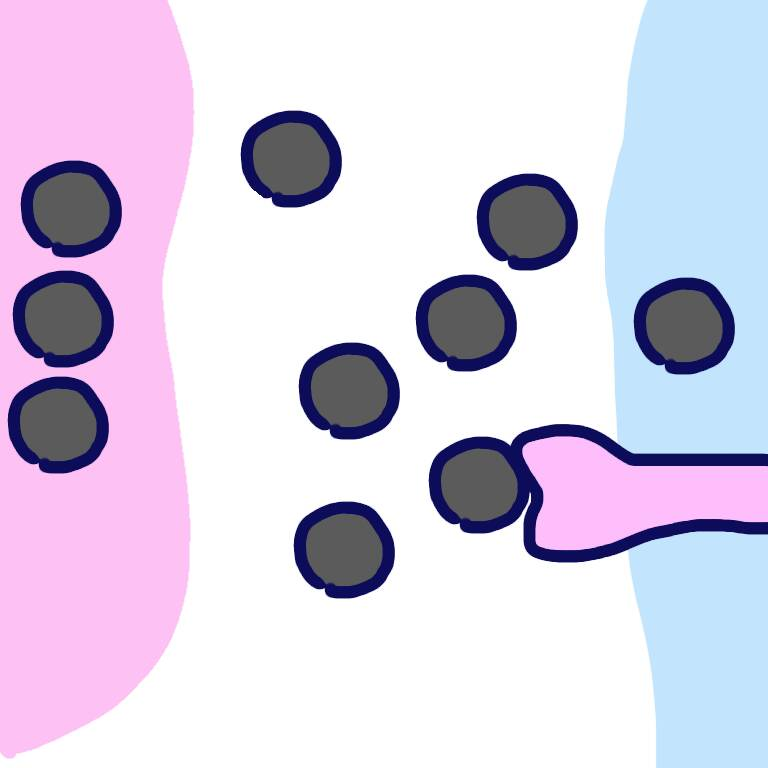# ExercisesHigeo

"Let's do the exercise for discovering new formula!"Pyon

"Oh...can I do well?"Penta

"It's no problem! Let's try!!"

## Basic Exercise for discover formulas1

Let's practice to find new formulas.

You don't have to solve problems, you only have to change some parts of the problem.

Think up new idea by yourself, please. Then, we will show you our idea.。

### Dies Game

Japan has a game long ago. The following game is a very traditional Japanese one.

You throw two dices, and if the sum of two is odd , it's called "Han". If the sum is else, it's called "Chyo".

Player must bet on "Han" or "Chyo". If the bet is correct, the player wins. If the player bet money, the players' betting money will be double.Let's change the rule of this game. First, please draw underline where you think you can change the original problem.

You throw two dices, and if the sum of two is odd , it's called "Han". If the sum is else, it's called "Chyo".

Player must bet on "Han" or "Chyo". If the bet is correct, the player wins. If the player bet money, the players' betting money will be double.

Which part of the problem you can change?

### Change numbers

The underlined part can be changed. For example, changing number of dice from two to three.

The game isn't changed greatly by this modification. However, it is meaningful to change.

You throw 3 dices, and if the sum of two is odd , it's called "Han". If the sum is else, it's called "Chyo".

Player must bet on "Han" or "Chyo". If the bet is correct, the player wins. If the player bet money, the players' betting money will be double.

Do you have another idea?

Next modification is great! This was proposed by a math teacher in Malaysia.

You throw3dices, and If the sum of the number is prime, we call it P. If not, we call it Q. Player must bet on "P" or "Q". If the bet is correct, the player wins. If the player bet money, the players' betting money will be double.

### Change the tool used in the game.

Do you have other idea?

In the following variant of the game, the situation will be changed greatly. In reality, this modification produces a mysterious phenomenon.

You draw two cards out of 52 cards, and if the sum of two is odd , it's called "Han". If the sum is else, it's called "Chyo". Player must bet on "Han" or "Chyo". If the bet is correct, the player wins. If the player bet money, the players' betting money will be double.

We denote two dices by A and B.

(i) In the following (a) and (b), the sum of A and B is even.

(a) When both of them are even. Then, the probability is 1/4.

(b) When both of them are odd. Then, the probability is1/4.

(ii) In the following (a) and (b), the sum of A and B is odd.

(a) When A is even and B is odd. Then, the probability is1/4.

(b) When B is even and A is odd. Then, the probability is1/4.

Therefore, when number of dice is two, the probability that the sum of A and B is even and the probability that it is odd are the same, because 1/4 + 1/4 = 1/2.

Next, we use three dices A, B, and C. Here we denote even by E and odd by O. Then, we have the following calculation.

(i) In the following (a), (b), (c) and (d), the sum of   four dices is even. Note that by EEE we mean the case that A is even, B is even, and C is even.)

(a) EEE Probability ; 1/8

(b) EOO Probability ; 1/8

(c) OEO Probability ; 1/8

(d) OOE Probability ; 1/8

(ii) In the following (a), (b), (c) and (d), the sum of   four dices is odd.

(a) OOO Probability ; 1/8

(b) EEO Probability ; 1/8

(c) OEE Probability ; 1/8

(d) EOE Probability ; 1/8

Therefore, when we have three dices, the probability of the sum of them is even and the probability of the sum is odd are equal by the calculation 1/2. 1/8 + 1/8 + 1/8 + 1/8 = 1/2.

If you use more than three dices, the situation is the same.

When we use three dices, there are 216 pattern since 6 * 6 * 6 = 216. Among the numbers that appear as the sum of three dices, we have prime numbers 3, 5, 7, 11, 13, and 17. The number of patterns that produce these prime numbers is 73.

Therefor, the probability of the sum is a prime number is 73/216 that is approximately l 0.337963.

Perhaps it will be interesting to do the same kind of calculation with more than three dices.

## Exercise 2

Now, let's change some parts of the original problem!

### Game of Nim

There are ten stones on a desk. Two players take turns and pick up within three stones. The player who takes up the last remaining stones or stone will be the winner of the game.

Which player have the winning strategy? What kind of strategy?Let's make a variant of the original problem. First, draw underlines where you are able to change the problem.

There is a pile of stones with 10 stones. Two players A and B take turns to pick up within 3 stones from one of two piles. The player who picks up the last remaining stones will be the winner. Which player has the winning strategy?

Could you come up with some ideas?

We choose red part. For example, changing number of coin other than 10 and changing number of coin which can be taken in a time.

There is a pile of stones with 10 stones. Two players A and B take turns to pick up within 3stones from one of two piles. The player who picks up the last remaining stones will be the winner. Which player has the winning strategy?

Did you think other idea? It's may be hard little.

We changed the player from two to three. The problem became hard.

There is a pile of stones with 10 stones.3 players A, B and C take turns to pick up within 3 stones from one of two piles. The player who picks up the last remaining stones will be the winner. Which player has the winning strategy?

Is there any other idea?

In the following problem, the underlined part can be changed from "winner" to "loser".

There is a pile of stones with 10 stones. Two players A and B take turns to pick up within 3 stones from one of two piles. The player who picks up the last remaining stones or a stone will be the loser. Which player has the winning strategy?

In the following problem, the underlined part can be changes. For example, the order of two player can be A,B,B,A,B,A,A,B,....

There is a pile of stones with 10 stones. Two players A and B take turns take turns to pick up within 3 stones from one of two piles. The player who picks up the last remaining stones will be the winner. Which player has the winning strategy?

Is there any other ideas? If you cannot propose a good idea, you may use a pile of stones with your own hands. It is sometimes good to use concrete objects with your hands.

There are two piles of stones. One with 10 stones and another with 9 stones. Two players A and B take turns to pick up within 3 stones from one of two piles. The player who picks up the last remaining stones will be the winner.

This type of problems are quite well known. These variants 1, 2,3,4,5,6 are also well known.

Answer. Let A and B be the first player and the second player. In this problem Player A has the winning strategy.

First, A takes two stones. If B picks up 1 or 2 or 3 stones, then A picks up 3 or 2 or 1 stones respectively. Then, 4 stones remain. If B picks up 1 or 2 or 3 stones, then A picks up 3 or 2 or 1 stones respectively. Then A is the winner.

The easiest way to modify the original problem is to change the number of stones.

variant１　　There is a pile of stones with 12 stones. Two players A and B take turns to pick up within 3 stones from one of two piles. The player who picks up the last remaining stones will be the winner.

Answer. Let A and B be the first player and the second player.

In this problem Player B has the winning strategy. If A picks up 1 or 2 or 3 stones, then B picks up 3 or 2 or 1 stones respectively. Then, 4 stones remain. If A picks up 1 or 2 or 3 stones, then B picks up 3 or 2 or 1 stones respectively. Then B is the winner.

As you see in these two previous problem, B wins the game if the number of stones is a multiple of the maximum number of stones to be picked up plus 1.

We can change the number of stones you can pick up at the same time.

variant2　　There is a pile of stones with 12 stones. Two players A and B take turns to pick up within 4 stones from one of two piles. The player who picks up the last remaining stones will be the winner.

Answer. Let A and B be the first player and the second player.

In this problem Player A has the winning strategy. First, A takes two stones. If B picks up 1 or 2 or 3 or 4 stones, then A picks up 4 or 3 or 2 or 2 stones respectively. Then, 5 stones remain. If B picks up 1 or 2 or 3 or 4 stones, then A picks up 4 or 3 or 2 or 1 stones respectively. Then A is the winner.

variant3　There is a pile of stones with 10 stones. Three players A, B and C take turns to pick up within 3 stones from one of two piles. The player who picks up the last remaining stones will be the winner. Which player has the winning strategy?

With three players, winning strategy became difficult. There is another problem. When you know that you are not going to win, then you can help one of your opponents to win the game. Which one to use?

variant4　There is a pile of stones with 10 stones. Two players A and B take turns to pick up within 3 stones from one of two piles. The player who picks up the last remaining stones or a stone will be the loser. Which player has the winning strategy?

The difference between this and previous one is the fact that you can win this game by making the number of remaining stones one.

variant6 There are two piles of stones. One with 10 stones and another with 9 stones. Two players A and B take turns to pick up within 3 stones from one of two piles. The player who picks up the last remaining stones will be the winner.

Answer. Let A and B be the first player and the second player.

In this problem Player A has the winning strategy. Player A picks up 1 stone from the pile of 10 stones. Then there are two piles with 9 stones. After that A have only to keep the numbers of two piles the same to win the game.